## Archimedean Tilings and Egyptian Fractions

#### February 5, 2012

Ever since I was a kid, I've loved Archimedean tilings of the plane: that is, tilings by regular polygons where all the edge lengths are the same and every vertex looks alike. Here's my favorite: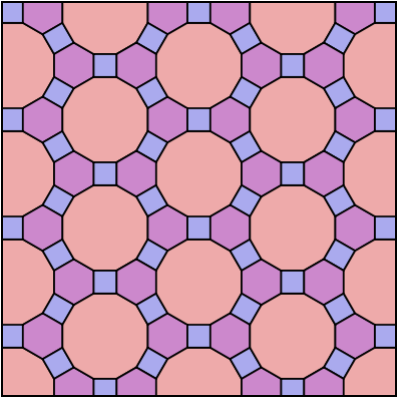There are also 11 others, two of which are mirror images of each other. But how do we know this? How do we list them all and be sure we haven't left any out?

The interior angle of a regular $$k$$-sided polygon is obviously $\displaystyle{\pi - \frac{2 \pi}{k}}$ since it's a bit less than 180 degrees, or $$\pi,$$ and how much? — well, $$1/k$$ times a full turn, or $$2 \pi.$$ But these $$\pi$$'s are getting annoying: it's easier to say 'a full turn' than write $$2\pi.$$ Then we can say the interior angle is $\displaystyle{\frac{1}{2} - \frac{1}{k}}$ times a full turn.

Now suppose we have an Archimedean tiling where $$n$$ polygons meet: one with $$k_1$$ sides, one with $$k_2$$ sides, and so on up to one with $$k_n$$ sides. Their interior angles must add up to a full turn. So, we have $\displaystyle{\left(\frac{1}{2} - \frac{1}{k_1}\right) + \cdots + \left(\frac{1}{2} - \frac{1}{k_n}\right) = 1 }$ or $\displaystyle{\frac{n}{2} - \frac{1}{k_1} - \cdots - \frac{1}{k_n} = 1}$ or $\displaystyle{ \frac{1}{k_1} + \cdots + \frac{1}{k_n} = \frac{n}{2} - 1 }$ So: to get an Archimedean tiling you need n whole numbers whose reciprocals add up to one less than n/2.

Looking for numbers like this is a weird little math puzzle. The Egyptians liked writing numbers as sums of reciprocals, so they might have enjoyed this game if they'd known it. The tiling I showed you comes from this solution: $\displaystyle{\frac{1}{4} + \frac{1}{6} + \frac{1}{12} = \frac{3}{2} - 1 }$ since it has 3 polygons meeting at each vertex: a 4-sided one, a 6-sided one and a 12-sided one.

Here's another solution:

$\displaystyle{\frac{1}{3} + \frac{1}{4} + \frac{1}{4} + \frac{1}{6} = \frac{4}{2} - 1 }$ It gives us this tiling: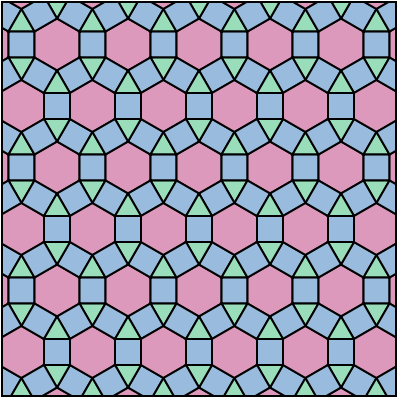Hmm, now I think this one is my favorite, because my eye sees it as a bunch of linked 12-sided polygons, sort of like chain mail. Different tilings make my eyes move over them in different ways, and this one has a very pleasant effect.

Here's another solution: $\displaystyle{\frac{1}{3} + \frac{1}{3} + \frac{1}{3} + \frac{1}{3} + \frac{1}{6} = \frac{5}{2} - 1 }$ This gives two Archimedean tilings that are mirror images of each other!

Of course, whether you count these as two different Archimedean tilings or just one depends on what rules you choose. And by the way, people usually don't say a tiling is Archimedean if all the polygons are the same, like this: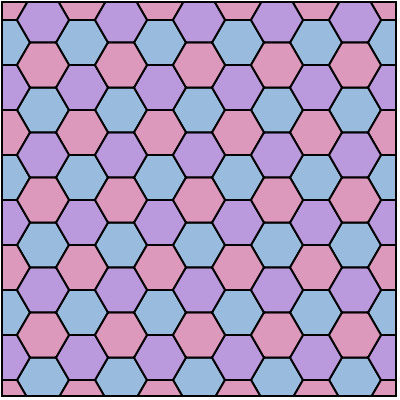They instead say it's regular. If modern mathematicians were inventing this subject, we'd say regular tilings are a special case of Archimedean tilings — but this math is all very old, and back then mathematicians treated special cases as not included in the general case. For example, the Greeks didn't even consider the number 1 to be a number!

So here's a fun puzzle: classify the Archimedean tilings! For starters, you need to find all ways to get $$n$$ whole numbers whose reciprocals add up to one less than $$n/2$$. That sounds hard, but luckily it's obvious that $n \le 6$ since an equilateral triangle has the smallest interior angle, of any regular polygon, and you can only fit 6 of them around a vertex. If you think a bit, you'll see this cuts the puzzle down to a finite search.

But you have to be careful, since there are some solutions that don't give Archimedean tilings. As usual, the number 5 causes problems. We have $\displaystyle{ \frac{1}{5} + \frac{1}{5} + \frac{1}{10} = \frac{3}{2} - 1 }$ but there's no way to tile the plane so that 2 regular pentagons and 1 regular decagon meet at each vertex! Kepler seems to have tried; here's a picture from his book Harmonices Mundi: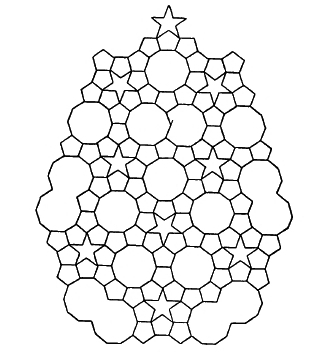It works beautifully at one vertex, but not for a tiling of the whole plane. To save the day he had to add some stars, and some of the decagons overlap! The Islamic tiling artists, and later Penrose, went further in this direction.

If you get stuck on this puzzle, you can find the answer here:

• Michal Krížek, Jakub Šolc, and Alena Šolcová, Is there a crystal lattice possessing five-fold symmetry?, AMS Notices 59 (January 2012), 22-30.

### Not enough?

In short, all Archimedean tilings of the plane arise from finding $$n$$ whole numbers whose reciprocals sum to $$n/2 - 1.$$ But what if the total is not enough? Don't feel bad: you might still get a tiling of the hyperbolic plane. For example, $\displaystyle{ \frac{1}{7} + \frac{1}{7} + \frac{1}{7} < \frac{3}{2} - 1 }$ so you can't tile the plane with 3 heptagons meeting at each corner... but you still get this tiling of the hyperbolic plane:which happens to be related to a wonderful thing called Klein's quartic curve.

You don't always win... but sometimes you do, so the game is worth playing. For example, $\displaystyle{ \frac{1}{3} + \frac{1}{3} + \frac{1}{3} + \frac{1}{3} + \frac{1}{3} + \frac{1}{4} < \frac{6}{2} - 1 }$ so you have a chance at a tiling of the hyperbolic plane where five equilateral triangles and a square meet at each vertex. And in this case, you luck out:For more beautiful pictures like these, see:

Uniform tilings in hyperbolic plane, Wikipedia.

• Don Hatch, Hyperbolic tesselations.

### Too much?

Similarly, if you've got $$n$$ reciprocals that add up to more than $$n/2 -1$$, you've got a chance at tiling the sphere. For example, $\displaystyle{ \frac{1}{3} + \frac{1}{3} + \frac{1}{3} + \frac{1}{3} + \frac{1}{5} > \frac{5}{2} - 1 }$ and in this case we luck out and get the snub dodecahedron. I thought it was rude to snub a dodecahedron, but apparently not:These tilings of the sphere are technically called Archimedean solids and (if all the polygons are the same) Platonic solids. Of these, only the snub dodecahedron and the 'snub cube' are different from their mirror images.

### Fancier stuff

In short, adding up reciprocals of whole numbers is related to Archimedean tilings of the plane, the sphere and the hyperbolic plane. But this is also how Egyptians would write fractions! In fact they even demanded that all the reciprocals be distinct, so instead of writing 2/3 as $$\frac{1}{3} + \frac{1}{3}$$, they'd write $$\frac{1}{2} + \frac{1}{6}.$$

It's a lousy system — doubtless this is why King Tut died so young. But forget about the restriction that the reciprocals be distinct: that's silly. If you can show that for every $$n > 1$$ the number $$4/n$$ can be written as $$1/a + 1/b + 1/c$$ for whole numbers $$a,b,c,$$ you'll be famous! So far people have 'only' shown it's true for $$n$$ up to a hundred trillion:

Erdös–Straus conjecture, Wikipedia.

So, see if you can do better! But a less stressful activity might be to read more about Egyptian fractions here:

• John Baez, 42.

As the title suggest, this focuses on the special magic arising from this equation: $\frac{1}{2} + \frac{1}{3} + \frac{1}{7} + \frac{1}{42} = 1$ However, near the end there's some stuff about Egyptian fractions in general, a kind of continuation of this page here.

And after that, you can read about Egyptian fractions, tilings and ADE classifications here:

• John Baez, This Week's Finds in Mathematical Physics (Week 182).

This only gets into 'Platonic' or 'regular' tilings, not the more general 'Archimedean' or 'semiregular' ones I'm talking about today — so the arithmetic works a bit differently.

In another direction, my colleague Julie Bergner has talked about how they Egyptian fractions show up in the study of 'groupoid cardinality':

• Julie Bergner, Groupoids and Egyptian fractions.

So, while nobody uses Egyptian fractions much anymore, they have a kind of eerie afterlife. For more on what the Egyptians actually did, try these:

• Ron Knott, Egyptian fractions.

Egyptian fractions, Wikipedia.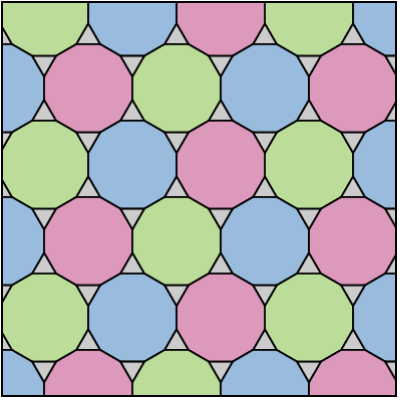$$\frac{1}{3} + \frac{1}{12} + \frac{1}{12} = \frac{3}{2} - 1$$

Text © 2013 John Baez
Images copyrighted by those who made them.
baez@math.removethis.ucr.andthis.edu